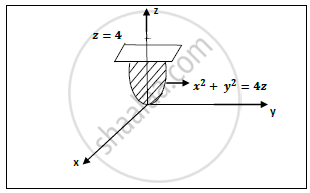# Find the Volume of the Paraboloid X 2 + Y 2 = 4 Z Cut off by the Plane 𝒛=𝟒 - Applied Mathematics 2

Sum

Find the volume of the paraboloid x^2+y^2=4z cut off by the plane 𝒛=𝟒

#### Solution

Paraboloid : x^2+y^2=4z Plane : 𝒛=𝟒Cartesian coordinate → cylindrical coordinates

(𝒙,𝒚,𝒛) → (𝒓,𝜽,𝒛)

Put 𝒙=𝒓𝒄𝒐𝒔 𝜽 ,𝒚=𝒓𝒔𝒊𝒏 𝜽 ,𝒛=𝒛  therefore x^2+y^2=r^2

∴ Paraboloid : r2 =4x and Plane : z = 4

If we are passing one arrow parallel to z axis from –ve to +ve we will get limits of z

therefore r^2/4≤ 𝒛 ≤ 𝟒
𝟎 ≤ 𝒓 ≤ 4

0 ≤ 𝜽 ≤ pi/2

Volume of given paraboloid cut off by the plane is given by ,

V = 4int_0^(pi/2) int_0^4 int_(r^2/4)^4rdrd theta dz

 = 4int_0^(pi/2) int_0^4 [4r-r^4/16]_(r^2/4)^4drd theta

 = 4int_0^(pi/2) int_0^4 [4r-r^3/4]drd theta

=4int_0^(pi/2)[2r^2-r^4/16]_0^4d theta

=4int_0^(pi/2)[32-16]d theta
𝑽 =𝟑𝟐 𝝅 cubic units

Concept: Triple Integration Definition and Evaluation
Is there an error in this question or solution?